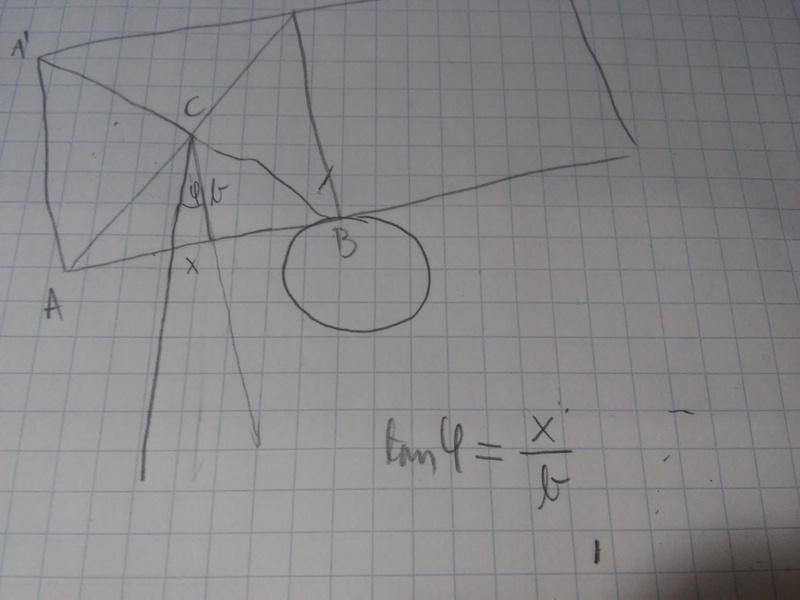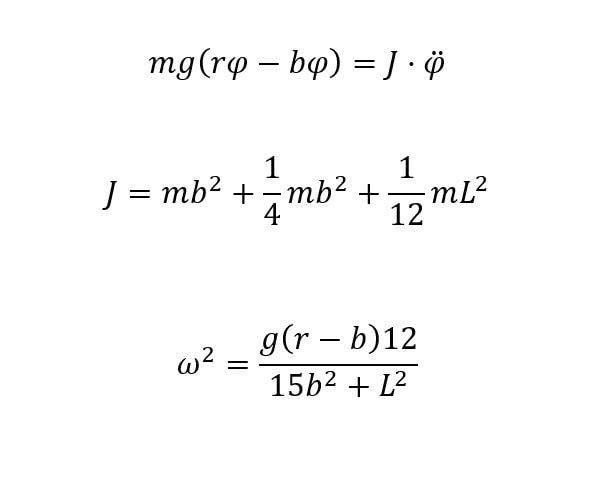# Finding frequency of a specific mechanical oscillator -- horizontal rod on pivotTSny
Homework Helper
Gold Member
OK, We are probably using the term "moment arm" differently. I think of it as the perpendicular distance from the axis of rotation to the line of action of the force. You might be thinking of it as just the distance from the axis to the point of application of the force or something else?

Ok, I know my previous attempt was wrong. You could think that I am just guessing the right equation, but no! Now I think that my answer is correct. The moment arm gets longer for b*sinφ, ok?

TSny
Homework Helper
Gold Member
The moment arm gets longer for b*sinφ, ok?
Yes, good.

On the other side it gets shorter for b*sinφ

TSny
Homework Helper
Gold Member
On the other side it gets shorter for b*sinφ
Yes.

But when talking about small φ that has no sense

TSny
Homework Helper
Gold Member
But when talking about small φ that has no sense
Why is that?

sin φ ≈ 0 for small φ

TSny
Homework Helper
Gold Member
sin φ ≈ 0 for small φ
That's going too far in the approximation.

I did the same approximation in the first part of my homework (calculating the oscillating time), when I say cos φ≈1 for small φ

TSny
Homework Helper
Gold Member
Keep terms of first order in φ. cosφ ≈ 1 - φ2/2 ≈ 1 to first order. What about sinφ?

sinφ=φ-(φ^3)/6

TSny
Homework Helper
Gold Member
sinφ=φ-(φ^3)/6
So, to first order in φ?

oh it is φ

TSny
Homework Helper
Gold Member
oh it is φ
That's right.

Well, I got that M_right - M_left = mg(rφ-bφ) ...M=torqueThe frequency gets smaller, as numerator gets smaller and denominator gets bigger. I hope it is correct! I would like to say you a big THANK YOU TSny!!! You helped me a lot... I learned a lot from solving this task with your help. I love this forum...

TSny
Homework Helper
Gold Member

ω2 is then negative, so there is no oscillation in real?

TSny
Homework Helper
Gold Member
ω2 is then negative, so there is no oscillation in real?
Yes, there is no oscillation. Looking back at your post#43, I think you need to add an overall negative sign to the first equation so that it's

##J \ddot{\phi} = - mg(r-b)\phi##.

If ##b > r## then you can see that ##\ddot{\phi} >0## if ##\phi## is given an initial small, positive value. So, the rod rotates away from the equilibrium position when it is released and there is no oscillation. Of course, the differential equation for ##\phi## breaks down as ##\phi## continues to increase since the differential equation was derived under the assumption that ##\phi## is always small.

Another way to investigate the general behavior of the system for various values of b is to derive the potential energy of the system as a function of ##\phi## without making a small angle approximation. Then plots of the potential energy function for various values of b will reveal a lot about the behavior. But that's probably for a rainy day.

•srecko97